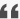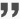# 新能源汽車角逐戰：新面孔、老玩家、資本局## 陪跑8年虧超41億元！馬云、史玉柱“割肉”華數傳媒

', "").replaceAll('

', ''); rest = rest1.replaceAll(/]*src=['"]([^'"]+)[^>]*>/gi, function (match, capture) { var url = capture.substr(0, 5); var url1 = capture.substr(0, 10); var url2 = capture.substr(capture.length - 5); var url3 = capture.substr(capture.length - 4, capture.length); if (url3 == "=gif" || url3 == "=jpg" || url3 == "=png" || url3 == "jpeg"||url3=="co=1") { capture = capture.substr(0, capture.length - 11) + ".jpg" } capture=capture.replace("jpeg","jpg"); capture=capture.replace("webp","jpg"); capture = capture.replace("http://img01.store.sogou.com/net/a/04/link?appid=100520029&url=", ""); if (url != "https") { if (url1 == "http://oss" || url1 == "http://img") { return ""; // return ""; } return "" } else if (url2 == "/webp") { capture = capture.substr(0, str.length - 5); var frameid = 'frameimg' + Math.random(); window['img'+index] = '